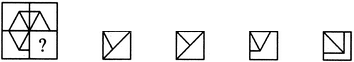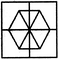# Non Verbal Reasoning - Pattern Completion - Discussion

### Discussion :: Pattern Completion - Section 1 (Q.No.46)

In each of the following questions, select a figure from amongst the four alternatives, which when placed in the blank space of figure (X) would complete the pattern.

46.

Identify the figure that completes the pattern.(X)                 (1)             (2)              (3)             (4)

 [A]. 1 [B]. 2 [C]. 3 [D]. 4

Explanation:Sachin said: (Jul 14, 2015) Right answer is D because in answer the bottom vertical line is straight and in given figure it is in pentagon figure type line.